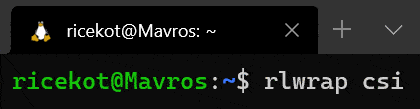I recently began reading “Structure and Interpretation of Computer Programs” as part of my “Learning Computer Science” project. I’m already enjoying it, and I’m going to be posting my notes and exercise solutions on this blog. Here’s some of the stuff I’ve read about until now.

### Applicative vs. Normal Order of Evaluation

Applicative Order Normal Order
“evaluate the arguments and then apply” “fully expand and then reduce”

MIT Scheme follows the applicative order of evaluation.

### Functions vs. Procedures

Functions Procedures
“what is” “how to”
e.g. “$y$ is the square root of $x$ if $y^2=x$. e.g. Heron’s method of computing square roots: $y_{n+1}=\frac{y_n+\frac{y_n}{x}}{2};\,\,n\in\mathbb{N}$ where $y_0$ is the initial guess. The value approximation $y_n$ of the square root of $x$ improves with each recursive step.

### Recursion and Iteration

Recursion Iteration
Recursion is a process that can be described as a chain of deferred operations. Iteration is a process that can be descibed completely, at any moment in time, by a fixed number of state variables.

There is a difference between a recursive process and a recursive procedure. A recursive procedure refers to the syntax of calling a procedure within its own definition. A process is called recursive based on how it evolves when it is executed.

I was quite surprised to learn that an iterative process can be generated by a recursive procedure. For example, consider the following two procedures that computer the factorial of a given positive integer.

(define (fact-recur n) (
(if (= n 1) 1
(* n (fact-recur (- n 1))))))

(define (fact-iter n) (
(define (iter product count) (
(if (> count n) product
(iter (* count product) (+ count 1)))))
(iter 1 1))


Both fact-recur and iter are recursive procedures. However, fact-iter will generate an iterative process on execution. Look at the solution of Exercise 1.9 below for a better understanding.

The implementation of a programming language is said to be tail-recursive if an iterative process described by a recursive procedure is executed as an iterative process. Scheme is a tail-recursive implementation. For other languages, iterative processes must be explicitly expressed with keywords like for, while, or do.

### My solutions to the first 10 exercises from Chapter 1

#### Exercise 1.1

10 12 8 3 6 a b 19 #f 4 16 6 16


#### Exercise 1.2

(/ (+ 5 4 (- 2 (- 3 (+ 6 (/ 4 5))))) (* 2 (- 6 2) (- 2 7)))


#### Exercise 1.3

(define (bruh a b c)
(if (> a b) (if (> b c) (sos a b) (sos a c))
(if (> a c) (sos a b) (sos b c))))

(define (sos x y) (+ (* x x) (* y y)))


#### Exercise 1.4

The procedure calculates a + b if b is positive and a - b if b is negative. In other words, it calculates a + |b|.

#### Exercise 1.5

With an interpreter that uses applicative-order evaluation, the expression cannot be evaluated. This is because the interpreter will get stuck when it tries to evaluate (p). (p) will evaluate to (p) and the interpreter will keep trying to simplify it with no luck.

An interpreter that uses normal-order evaluation will never encounter (p) and the program will return 0.

#### Exercise 1.6

The interpreter will get stuck in an infinite recursive loop and nothing will be returned. This is because scheme uses applicative-order evaluation. This means that the arguments of a procedure are evaluated before the procedure is applied. Since new-if is a procedure and not a special form, the interpreter calls sqrt-iter each time it tries to evaluate the else-clause of new-if. As a result, new-if is never applied and guess is never returned even if it is good-enough?

#### Exercise 1.7

The guess is considered good enough if the improved guess is within 0.1% of the original guess.

(define (good-enough? guess x)
(< (abs (- guess (improve guess x))) (* 0.001 guess)))


#### Exercise 1.8

(define (cbrt-iter old-guess guess x)
(if (cbrt-good-enough? old-guess guess) guess
(cbrt-iter guess
(cbrt-improve guess x)
x)))

(define (cbrt-improve guess x)
(/ (+ (/ x (* guess guess)) (* 2 guess)) 3))

(define (cbrt-good-enough? old-guess guess)
(< (abs (- old-guess guess)) (* 0.001 old-guess)))

(define (cbrt x)
(cbrt-iter 0 1.0 x))


#### Exercise 1.9

The first procedure generates a recursive process.

(+ 4 5)
(inc (+ 3 5))
(inc (inc (+ 2 5)))
(inc (inc (inc (+ 1 5))))
(inc (inc (inc (inc (+ 0 5)))))
(inc (inc (inc (inc 5))))
(inc (inc (inc 6)))
(inc (inc 7))
(inc 8)
9


The second procedure generates an iterative process.

(+ 4 5)
(+ 3 6)
(+ 2 7)
(+ 1 8)
(+ 0 9)
9


#### Exercise 1.10

(A 1 0) = 1024
(A 2 4) = 65536
(A 3 3) = 65536

$f(n)=2n$

$g(n)={\left\lbrace\begin{matrix}{0}&{\quad\text{if}\quad}{n}={0}\\ 2^n&{\quad\text{if}\quad}{n}\ge{1}\end{matrix}\right.}$ $h(n)={\left\lbrace\begin{matrix}{0}&{\quad\text{if}\quad}{n}={0}\\ 2&{\quad\text{if}\quad}{n}={1} \\ 2^{h(n-1)}&{\quad\text{if}\quad}{n}\ge{2}\end{matrix}\right.}$

### My Scheme Setup

It’s irrelevant but, I’m using CHICKEN on WSL.Until next time!